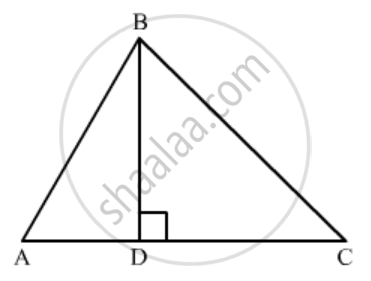Advertisement Remove all ads

# In ∆Abc, If Bd ⊥ Ac and Bc2 = 2 Ac . Cd, Then Prove that Ab = Ac. - Mathematics

Sum

In ∆ABC, if BD ⊥ AC and BC2 = 2 AC . CD, then prove that AB = AC.

Advertisement Remove all ads

#### SolutionSince Δ ADB  is right triangle right angled at D

AB^2=AD^2+BD^2

In right Δ BDC, we have

CD^2+BD^2=BC^2

Sine 2AC.DC=BC^2

⇒DC^2+BD^2=2AC.DC

2AC.DC=AC^2-AC^2+DC^2+BD^2

AC^2=AC^2+DC^2-2AC.DC+BD^2

AC^2=(AC-DC)^2+BD^2

AC^2=AD^2+BD^2

Now substitute AD^2+BD^2=AB^2

AC^2=AB^2

AC=AB

Concept: Triangles Examples and Solutions
Is there an error in this question or solution?
Advertisement Remove all ads

#### APPEARS IN

RD Sharma Class 10 Maths
Chapter 7 Triangles
Q 30 | Page 127
Advertisement Remove all ads

#### Video TutorialsVIEW ALL 

Advertisement Remove all ads
Share
Notifications

View all notifications

Forgot password?
Course